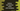# Python id() function explanation with examples## Learn how Python id() function works with examples:

The id function is used to get the identity of an object. It returns one integer value which is a unique value and constant value for that object. We may get unique ids if two objects have a non-overlapping lifetime.

### Syntax of id() function:

The syntax of id() function is:

``id(object)``

We need to pass the object and it returns the identity of the object, which is an integer value.

Other than objects, we can also pass a string, list, tuple, integer etc. to this function.

### Example of id() function with numbers:

The below program shows how the id() function works with numbers:

``````x = 10
y = 20
z = 10
x1 = x

print('id of x: ',id(x))
print('id of y: ',id(y))
print('id of z: ',id(z))
print('id of x1: ',id(x1))``````

It will print:

``````id of x:  2347957709392
id of y:  2347957709712
id of z:  2347957709392
id of x1:  2347957709392``````

As you can see here, the id() function returns different numbers as the ids. The ids of x, z and x1 are the same because these variables are equal i.e. 10.

### Example of id() function with strings:

The below program shows how the id() function works with different strings:

``````str1 = 'hello'
str2 = 'world'
str3 = 'hello'
str4 = str2

print('id of str1: ',id(str1))
print('id of str2: ',id(str2))
print('id of str3: ',id(str3))
print('id of str4: ',id(str4))``````

It will print:

``````id of str1:  3068235986800
id of str2:  3068235897968
id of str3:  3068235986800
id of str4:  3068235897968``````
• The ids of str1 and str3 are equal because the strings are the same.
• The ids of str2 and str4 are equal because both are referring to the same string.

### Example of id() function with list:

Let’s use it with lists:

``````list1 = ['hello', 'world']
list2 = ['hello', 'world']
list3 = ['hello']

print('id of list1: ',id(list1))
print('id of list2: ',id(list2))
print('id of list3: ',id(list3))``````

Even though the contents of list1 and list2 are the same, it will produce different ids for both.

``````id of list1:  2280471356352
id of list2:  2280471644992
id of list3:  2280471706816``````

### Example of id() function with custom objects:

We can use the id() function with custom objects. It will produce different ids even if the content of the objects is similar.

``````class Student:
name = 'Alex'

std1 = Student()
std2 = Student()
std3 = std1

print('id of std1: ',id(std1))
print('id of std2: ',id(std2))
print('id of std3: ',id(std3))``````

In this example, the Student class has only one property name, which is equal to ‘Alex’.

If you run this program, it will print:

``````id of std1:  1538234523264
id of std2:  1538234523216
id of std3:  1538234523264``````

The ids of std1 and std2 are different. Since both std3 and std1 are referring to the same objects, the ids are the same for these two.